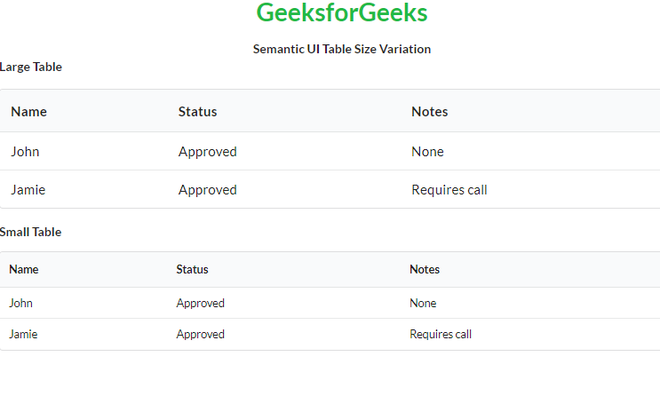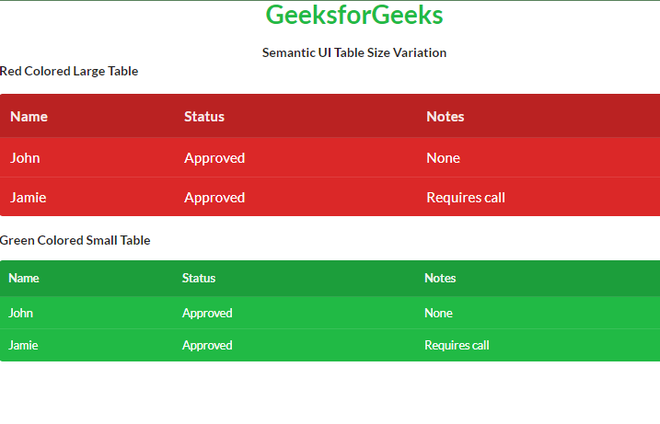Open in App
Not now

# Semantic-UI Table Size Variation

• Last Updated : 14 Mar, 2022

Semantic UI is an open-source framework that uses CSS and jQuery to build great user interfaces. It is the same as a bootstrap for use and has great different elements to use to make your website look more amazing. It uses a class to add CSS to the elements.

Tables are an easy way to organize a lot of data. A table is an arrangement of data in rows and columns, or possibly in a more complex structure. Tables are widely used in communication, research, and data analysis. Tables are useful for various tasks such as presenting text information and numerical data. It can be used to compare two or more items in the tabular form layout. Tables are used to create databases. An HTML table and a Semantic UI table both are the same structurally.

Using Table Size Variation we can set the size of our table to either large or small.

Semantic UI Table Size Variation Classes:

• small: This class creates a table of small size.
• large: This class creates a table of large size.

Syntax:

```<table class="ui size-class table">
<tr>
<td></td>
...
</tr>
...
</table>```

Example 1: This is a basic example illustrating Table Size Variation made using Semantic UI.

## HTML

 ```<``html``>`` ` `<``head``>``    ``<``title``>Semantic UI Table Size Variation`` ` `    ``<``link` `href``=``"https://cdnjs.cloudflare.com/ajax/libs/semantic-ui/2.4.1/semantic.min.css"``          ``rel``=``"stylesheet"``/>`` ` `    ``<``script` `src``=``"https://code.jquery.com/jquery-3.1.1.min.js"` `integrity``=``"sha256-hVVnYaiADRTO2PzUGmuLJr8BLUSjGIZsDYGmIJLv2b8="` `crossorigin``=``"anonymous"``> ``    ````     ` `    ``<``script` `src``=``"https://cdnjs.cloudflare.com/ajax/libs/semantic-ui/2.4.1/semantic.min.js"``>``    `````` ` `<``body``>``    ``<``center``>``        ``<``h1` `class``=``"ui green header"``>GeeksforGeeks``        ``<``strong``>Semantic UI Table Size Variation``    ```` ` `    ``<``b``>Large Table``    ``<``table` `class``=``"ui large table"``>``        ``<``thead``>``            ``<``tr``>``            ``<``th``>Name``            ``<``th``>Status``            ``<``th``>Notes``            ````        ````        ``<``tbody``>``            ``<``tr``>``            ``<``td``>John``            ``<``td``>Approved``            ``<``td``>None``            ````            ``<``tr``>``            ``<``td``>Jamie``            ``<``td``>Approved``            ``<``td``>Requires call``            ````        ````    ```` ` `    ``<``b``>Small Table``    ``<``table` `class``=``"ui small table"``>``        ``<``thead``>``            ``<``tr``>``            ``<``th``>Name``            ``<``th``>Status``            ``<``th``>Notes``            ````        ````        ``<``tbody``>``            ``<``tr``>``            ``<``td``>John``            ``<``td``>Approved``            ``<``td``>None``            ````            ``<``tr``>``            ``<``td``>Jamie``            ``<``td``>Approved``            ``<``td``>Requires call``            ````        ````    `````` ` ``

Output:Semantic UI Table Size Variation

Example 2: This is a basic example illustrating different colors of Table Size Variation made using Semantic UI.

## HTML

 ```<``html``>`` ` `<``head``>``    ``<``title``>Semantic UI Table Size Variation`` ` `    ``<``link` `href``=``"https://cdnjs.cloudflare.com/ajax/libs/semantic-ui/2.4.1/semantic.min.css"``          ``rel``=``"stylesheet"``/>`` ` `    ``<``script` `src``=``"https://code.jquery.com/jquery-3.1.1.min.js"` `integrity``=``"sha256-hVVnYaiADRTO2PzUGmuLJr8BLUSjGIZsDYGmIJLv2b8="` `            ``crossorigin``=``"anonymous"``> ``    ```` ` `    ``<``script` `src``=``"https://cdnjs.cloudflare.com/ajax/libs/semantic-ui/2.4.1/semantic.min.js"``>``    `````` ` `<``body``>``    ``<``center``>``        ``<``h1` `class``=``"ui green header"``>GeeksforGeeks``        ``<``strong``>Semantic UI Table Size Variation``    ````    ``<``b``>Red Colored Large Table``    ``<``table` `class``=``"ui large table inverted red"``>``        ``<``thead``>``            ``<``tr``>``            ``<``th``>Name``            ``<``th``>Status``            ``<``th``>Notes``            ````        ````        ``<``tbody``>``            ``<``tr``>``            ``<``td``>John``            ``<``td``>Approved``            ``<``td``>None``            ````            ``<``tr``>``            ``<``td``>Jamie``            ``<``td``>Approved``            ``<``td``>Requires call``            ````        ````    ```` ` `    ``<``b``>Green Colored Small Table``    ``<``table` `class``=``"ui small table inverted green"``>``        ``<``thead``>``            ``<``tr``>``            ``<``th``>Name``            ``<``th``>Status``            ``<``th``>Notes``            ````        ````        ``<``tbody``>``            ``<``tr``>``            ``<``td``>John``            ``<``td``>Approved``            ``<``td``>None``            ````            ``<``tr``>``            ``<``td``>Jamie``            ``<``td``>Approved``            ``<``td``>Requires call``            ````        ````    `````` ` ``

Output:Semantic UI Table Size Variation

My Personal Notes arrow_drop_up